# Overlapping Histograms with Matplotlib in Python

• Last Updated : 26 Nov, 2020

Histograms are a way of visualizing the data. Here, we will learn how to plot overlapping histograms in python using Matplotlib library. matplotlib.pyplot.hist() is used for making histograms.

Let’s take the iris dataset and plot various overlapping histograms with Matplotlib.

Step 1: Import the libraries

## Python3

 `# importing libraries``import` `matplotlib.pyplot as plt``import` `seaborn as sns`

## Python3

 `# load the iris dataset``data ``=` `sns.load_dataset(``'iris'``)`` ` `# view the dataset``print``(data.head(``5``))`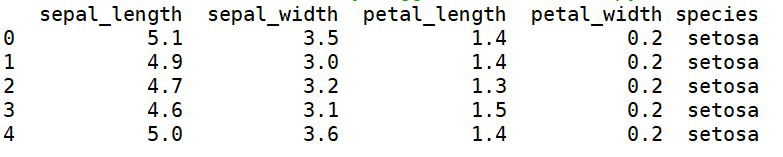Step 3: Let us plot histograms for sepal_length and petal_length.

## Python3

 `# plotting histograms``plt.hist(data[``'petal_length'``], ``         ``label``=``'petal_length'``)`` ` `plt.hist(data[``'sepal_length'``], ``         ``label``=``'sepal_length'``)`` ` `plt.legend(loc``=``'upper right'``)``plt.title(``'Overlapping'``)``plt.show()`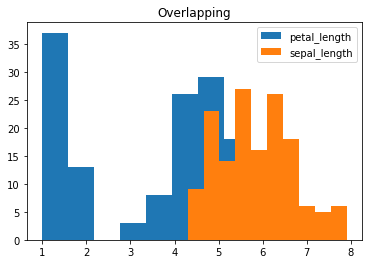Here, we can see that some part of the histogram for ‘petal_length’ has been hidden behind the histogram for ‘sepal_length’. To properly visualize both the histograms, we need to set the transparency parameter, alpha to a suitable value. So let’s check various values for alpha and find out the suitable one.

Step 4: Set alpha=0.5 for both sepal_length and petal_length

## Python3

 `plt.hist(data[``'petal_length'``], ``         ``alpha``=``0.5``, ``# the transaparency parameter``         ``label``=``'petal_length'``)`` ` `plt.hist(data[``'sepal_length'``],``         ``alpha``=``0.5``,``         ``label``=``'sepal_length'``)`` ` `plt.legend(loc``=``'upper right'``)``plt.title(``'Overlapping with both alpha=0.5'``)``plt.show()`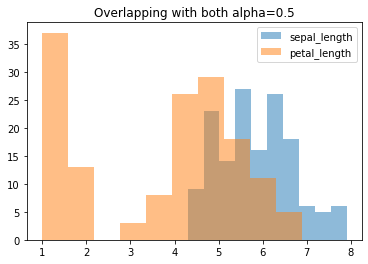Step 5: Set alpha=0.1 for sepal_length and 0.9 for petal_length

## Python3

 `plt.hist(data[``'petal_length'``], ``         ``alpha``=``0.9``,``         ``label``=``'petal_length'``)`` ` `plt.hist(data[``'sepal_length'``],``         ``alpha``=``0.1``,``         ``label``=``'sepal_length'``)`` ` `plt.legend(loc``=``'upper right'``)``plt.title(``'Overlapping with alpha=0.1 and 0.9 for sepal and petal'``)``plt.show()`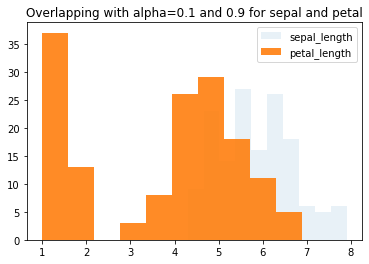Through the above two steps, we can infer that for a better visualization for both the histograms, alpha=0.5 would be the most suitable option for the transparency parameter.

Now, to plot more than two overlapping histograms where we need custom colors, let’s follow step 6.

Step 6: Create more than 2 overlapping histograms with customized colors.

## Python3

 `# plotting more than 2 overlapping histograms``plt.hist(data[``'sepal_width'``], ``         ``alpha``=``0.5``, ``         ``label``=``'sepal_width'``,``         ``color``=``'red'``) ``# customized color parameter`` ` `plt.hist(data[``'petal_width'``], ``         ``alpha``=``0.5``,``         ``label``=``'petal_width'``,``         ``color``=``'green'``)`` ` `plt.hist(data[``'petal_length'``], ``         ``alpha``=``0.5``,``         ``label``=``'petal_length'``,``         ``color``=``'yellow'``)`` ` `plt.hist(data[``'sepal_length'``], ``         ``alpha``=``0.5``,``         ``label``=``'sepal_length'``,``         ``color``=``'purple'``)`` ` `plt.legend(loc``=``'upper right'``)``plt.show()`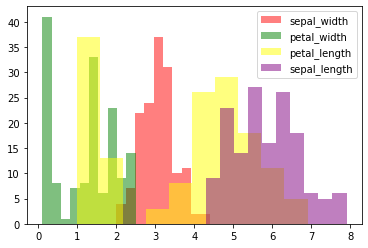Thus, in this article, we learned how to plot overlapping histograms using Matplotlib, how to set their transparency values, and customize their colors.

My Personal Notes arrow_drop_up